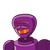# (y+y^3/3+x^2/2) dx+ 1/4(x+xy^2). Solve the differential equation.​

(y+y^3/3+x^2/2) dx+ 1/4(x+xy^2). Solve the differential equation.​

### 1 thought on “(y+y^3/3+x^2/2) dx+ 1/4(x+xy^2). Solve the differential equation.​”

1.